## 公告

《如何自學程式設計》入選 Google Play 台灣地區2016年度最佳書籍｜本站於 Google Play 銷售電子書。

------
Udemy ｜ Python 專案開發入門的二十一堂課 | 促銷優惠

### Swift 入門指南 V1.00 - 單元 6 - 迴圈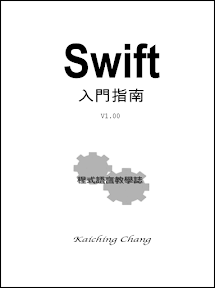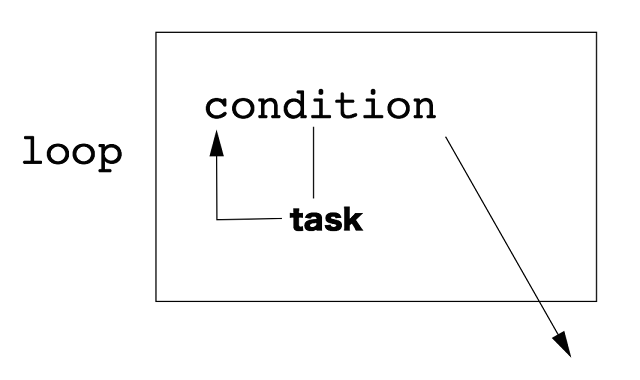Swift 中有四種迴圈，分別是 while 迴圈 (while loop) 、 do-while 迴圈 (do-while loop) 、 for 迴圈 (for loop) 及 for-in 迴圈 (for-in loop) ，四種迴圈可以互相替代，端視自己喜歡用哪一種囉！我們先來看看 while 迴圈的寫法

 var i1 = 0 // 設定控制變數 var sum1 = 0 while i1 <= 10 { // 條件 // 迴圈工作區 sum1 += i1 i1++ // 調整控制變數值 } println(sum1)

• 設定控制變數
• 條件
• 調整控制變數值

while 迴圈的控制變數 (control variable) 必須在 while 之前就先設定好，此例中將控制變數 i1 設定為 10 。然後進入 while 的地方，條件 (condition) 就在 while 之後的運算式 (expression) 中，此例的條件為當控制變數 i1 小於 10 時，迴圈便會重複執行。迴圈工作區，也就是 while 之後用大括弧圍住的程式區塊，這裡，我們只有簡單的印出控制變數 i1 的值，另外，最後需要有調整控制變數值的地方。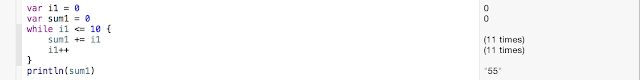do-while 迴圈是先進行迴圈工作的第一次，工作結束後才進行條件檢查，我們將上例用 do-while 改寫，如下

 var i2 = 0 var sum2 = 0 do { sum2 += i2 i2++ } while i2 <= 10 println(sum2)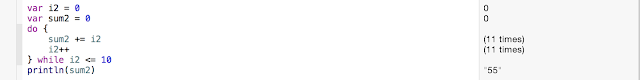for 迴圈則是把控制變數的設定、迴圈結束條件、控制變數的調整全都寫在 for 之後，如下

 var i3: Int var sum3 = 0 for i3 = 0; i3 <= 10; i3++ { sum3 += i3 } println(sum3)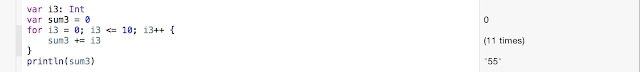for-in 迴圈用於集合體型態 (collection type) ，例如

 var i4: Int var sum4 = 0 for i4 in 0...10 { sum4 += i4 } println(sum4)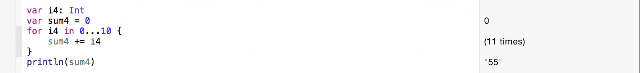while true { // 迴圈工作區 }

 while true { if word == "quit" { break } // 迴圈工作區 }

## 中英文術語對照

 程式 program 迴圈 loop 區塊 block while 迴圈 while loop do-while 迴圈 do-while loop for 迴圈 for loop for-in 迴圈 for-in loop 控制變數 control variable 條件 condition 運算式 expression 集合體型態 collection type 無窮迴圈 infinite loop